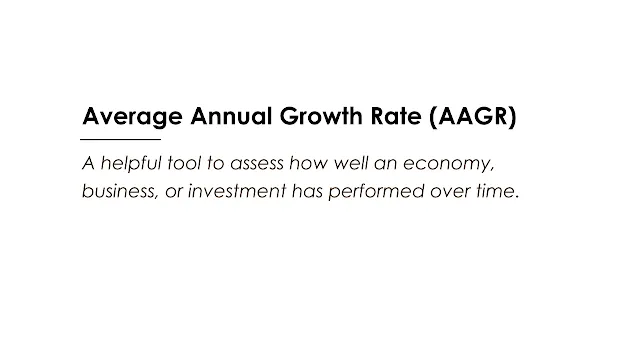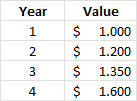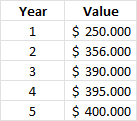# Average Annual Growth Rate (AAGR)Image: Moneybestpal.com

### The average annual growth rate (AAGR) is a helpful tool to assess how well an economy, business, or investment has performed over time. It displays the typical annual percentage change in a variable's value. In this blog article, we will define the AAGR, demonstrate its calculation, and provide an example-based explanation of how to use it.

#### What is the AAGR?

The arithmetic average annual growth rate (AAGR) is the average growth rate over a period of time. To offer investors an indication of the direction and size of the change, it can be applied to any financial metric, including revenue, profit, cash flow, expenses, etc.

The AAGR makes the assumption that the growth rate will remain constant over time because it does not account for the impacts of compounding. It is therefore a straightforward and linear measure, but it is also less precise than the compound annual growth rate (CAGR), which takes the overall impact of growth over time into consideration.

Any investment can have its AAGR calculated, but it will not include any information about how risky the investment overall is as determined by price volatility. As a result, it should be utilized cautiously and in conjunction with other performance and risk indicators.

#### How to calculate the AAGR?

The formula for the AAGR is:

AAGR = (GR1 + GR2 + ... + GRn) / n

Where:
• GR1 = Growth rate in period 1
• GR2 = Growth rate in period 2
• GRn = Growth rate in period n
• n = Number of periods

To calculate the growth rate for each period, we use the following formula:

GR = (EV / BV) - 1

Where:
• EV = Ending value
• BV = Beginning value

One thing to keep in mind is that the periods used should all be of equal length—for instance, years, months, or weeks—and not mix periods of different duration.

#### How to interpret the AAGR?

The AAGR informs us of the growth or average annual return over a number of periods. It can be used to compare the long-term performance of various assets, companies, or economies. However, it has some limitations that should be considered:
• The AAGR does not capture the erratic or volatile growth rates over time. It might conceal some variations or anomalies that could have an impact on performance as a whole.
• The AAGR does not take the effects of compounding into account, hence the actual growth over time may be underestimated or overestimated. For instance, if investment rises by 10% in year 1 and by 20% in year 2, the AAGR is 15%, but the CAGR is 14.87%, which represents the fact that the second year's growth is based on a greater base value than the first year's.
• The selection of endpoints or periods may affect the AAGR. For instance, depending on how the values vary over time, calculating the AAGR for an investment from years 1 to 5 may yield a different answer than calculating it from years 2 to 6.
To obtain a more complete picture of the investment, in addition to using the AAGR, we need also consider other performance and risk indicators, such as the CAGR, the standard deviation, the Sharpe ratio, etc.

#### Examples of AAGR

Let's look at some examples of how to calculate and interpret the AAGR.

#### Example 1: Financial Investment

Assume that an investment has the following values over the course of four years:Image: Moneybestpal.com

To calculate the AAGR, we first need to calculate the growth rate for each year:
• Year 1 growth = N/A
• Year 2 growth = (\$1200 / \$1000) - 1 = 20%
• Year 3 growth = (\$1350 / \$1200) - 1 = 12.5%
• Year 4 growth = (\$1600 / \$1350) - 1 = 18.52%
Then, we add up the growth rates and divide by 3 (the number of periods):

AAGR = (20% + 12.5% + 18.52%) / 3 = 17.01%

This means that on average, the investment grew by 17.01% per year over the four-year period.

Assume that a business has the following annual revenues for the past five years:Image: Moneybestpal.com

To calculate the AAGR, we first need to calculate the growth rate for each year:
• Year 1 growth = N/A
• Year 2 growth = (\$356,000 / \$250,000) - 1 = 42.4%
• Year 3 growth = (\$390,000 / \$356,000) - 1 = 9.55%
• Year 4 growth = (\$395,000 / \$390,000) - 1 = 1.28%
• Year 5 growth = (\$400,000 / \$395,000) - 1 = 1.27%

Then, we add up the growth rates and divide by 4 (the number of periods):

AAGR = (42.4% + 9.55% + 1.28% + 1.27%) / 4 = 13.63%

This means that on average, the business revenue grew by 13.63% per year over the five-year period.

#### Conclusion

The average annual growth rate (AAGR) is a straightforward and linear indicator of how much a variable's value has changed on average over time. It can be used to assess how various investments, companies, or economies have fared over time. However, there are several drawbacks that should be taken into mind, such as the fact that it does not take the impacts of compounding or the fluctuation of growth rates over time into account. As a result, it should be utilized cautiously and in conjunction with other performance and risk indicators.

Tags